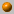##### Personal tools
You are here: Home Computational Geometry - Fall 2015/2016
« February 2023 »
February
SuMoTuWeThFrSa
1234
567891011
12131415161718
19202122232425
262728

# Computational Geometry - Fall 2015/2016

Instructor: Dan Halperin , danha AT post

Grader: Israela Solomon, israela5 AT mail.tau.ac.il
mailbox 305, Schreiber, 2nd floor

Monday, 16:00--19:00

Room: Orenstein 111

Office hours: Monday, 19:00--20:00

The course covers fundamental algorithms for solving geometric problems such as computing convex hulls, intersection of line segments, Voronoi diagrams, polygon triangulation, and linear programming in low dimensional space. We will also discuss several applications of geometric algorithms to solving problems in robotics, GIS (geographic information systems), computer graphics, and more.

### Bibliography:

The main textbook of the course is:

Computational Geometry: Algorithms and Applications (CGAA), 2nd or 3rd edition by M. de Berg, M. van Kreveld, M. Overmars, and O. Schwarzkopf.

A bibliographic list for the course

### Slides:

I will often use slides that accompany the main textbook of the course is.The slides are by Marc van Kreveld and they can be found

at the following site: Geometric Algorithms

The standard assignments will account for 10% of the final grade, in case they improve the final grade.
The grade in the final exam, to be held on Friday, January 29th, 2016, will account for the remaining 90%
of the grade in the course. Although the assignment grades will be used only for improving the final grade,
submission of the assignments is mandatory and a prerequisite for taking the exam.

Exam(ple), pdf file

About halfway through the course, we will present a programming assignment.
We encourage you to submit the programming assignment as well. This is not mandatory.
If however you do submit it, then your grade breakdown will be 10% the standard assignments,
15% the programming assignment, and 75% the final exam. Here as well,
the assignments grades will be counted toward the final grade only if they improve it.

• Assignment no. 1 (pdf file), due: November 9th, 2015. Another hint for Exercise 1.1(b) will be given at the lesson on November 2nd.
• Assignment no. 2 (pdf file), due: November 23rd, 2015.
• Assignment no. 3 (pdf file), due: December 14th, 2015.
• Self-study work: Describe in full detail the 3D analog of the 2D algorithm for Linear Programming that we studied in class, including the unbounded case.
• Programming Assignment (pdf file), due: January 7th, 2016 (you may work and submit in pairs). Additional information.
• Assignment no. 4 (pdf file), due: January 4th, 2016.

### Course Summary:

Below you'll find a very brief summary of what was covered in class during the semester.
This should not be taken as a complete description of the course's contents.
For an outline of the course, see for example, the course summary in the 2010 computational geometry course.19.10.15
What is Computational Geometry? Course outline, motivation and techniques. Naive convex hull algorithms (see Chapter 3 in O'Rourke's book). Orientation (Side-of-line) test. Gift wrapping. Graham's O(n log n) algorithm (Chapter 1 in CGAA).26.10.15
Lower bound for convex hull algorithms. Output sensitive algorithm to compute the intersections of line segments: Bentley Ottmann's plane sweep. The doubly-connected edge list (DCEL)(CGAA, Chapter 2).2.11.15

The doubly-connected edge list (DCEL)and map overlay (CGAA, Chapter 2). The Douglas Peucker simplification algorithm.9.11.15

Polygon triangulation. Art gallery problems. The material covered in this lesson could be found in O'Rourke's book, Chapters 1 and 2, and in CGAA, Chapter 3.

We have shown that \Omega(n^{3/2} guards are sometimes needed to guard a polyhedron with n vertices. The construction is due to Seidel and its presentation is taken from the book Art Gallery Theorems and Algorithms by J. O'Rourke.16.11.15

The casting problem. Half plane intersection. Introduction to Linear Programming (CGAA, Chapter 4).23.11.15

Linear Programming continued. The unbounded case in 2D. The minimum enclosing disk problem (CGAA, Chapter 4).30.11.15

Orthogonal range searching, kd-trees, range-trees (CGAA, Chapter 5).7.12.15

Introduction to CGAL, presented by Efi Fogel [slides].14.12.15

Trapezoidal maps and planar point location (CGAA, Chapter 6).21.12.15

Voronoi diagrams of points in the plane: structure, complexity, and Fortune's algorithm (CGAA, Chapter 7).28.12.15

Point-Line duality, arrangements of lines in the plane, the zone theorem and incremental construction of the arrangement (CGAA, Chapter 8). The minimum area triangle problem (CGAL Arrangements and Their Applications, Section 4.2.1).

Optimal randomized incremental construction for guaranteed logarithmic planar point location, presented by Michal Kleinbort [slides].4.1.16

Point-line duality continued.
Multi robot motion planning, presented by Kiril Solovey [slides]11.1.16

Delaunay triangulations (CGAA, Chapter 9).
Summary of the programming assignment, presented by Efi Fogel. See also Programming assignment web page.

##### Document Actions